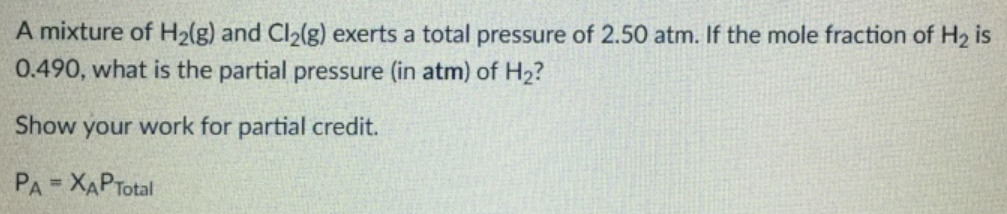# Problem: A mixture of H2(g) and Cl2(g) exerts a total pressure of 2.50 atm. If the mole fraction of H2 is 0.490, what is the partial pressure (in atm) of H2? Show your work for partial credit. PA = XAPTotal

🤓 Based on our data, we think this question is relevant for Professor Delgado's class at FIU.

###### FREE Expert Solution###### Problem Details

A mixture of H2(g) and Cl2(g) exerts a total pressure of 2.50 atm. If the mole fraction of H2 is 0.490, what is the partial pressure (in atm) of H2? Show your work for partial credit. P= XAPTotal